RS Aggarwal Class 8 Solutions Chapter 24 - Probability

RS Aggarwal Class 8 Chapter 24 - Probability Solutions Free PDF

RS Aggarwal class 8 solutions for chapter 24 probability are provided here for free. Before solving the questions from probability let us learn what is a probability. Probability is the measure of the likelihood that an event will occur. In other words, the probability is the analysis of random phenomena. As we all know practice is the key to success. To practice questions based on probability students are advised to solve questions from RS Agarwal for class 8.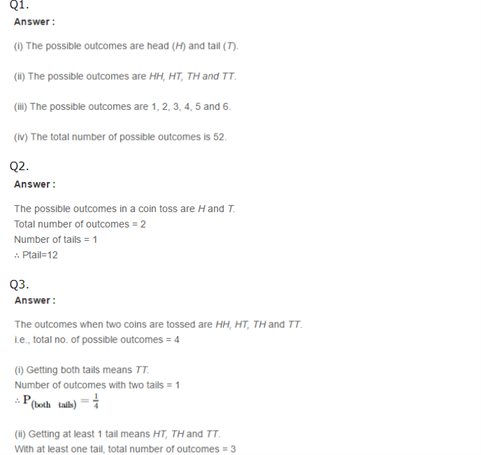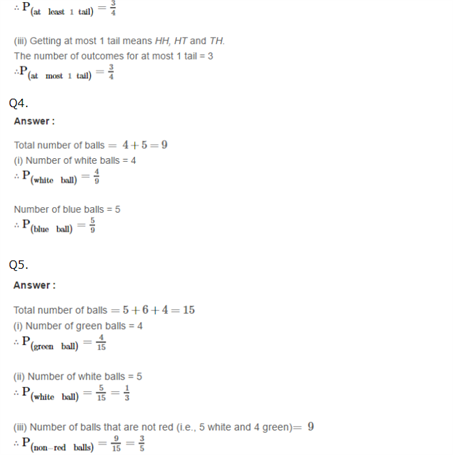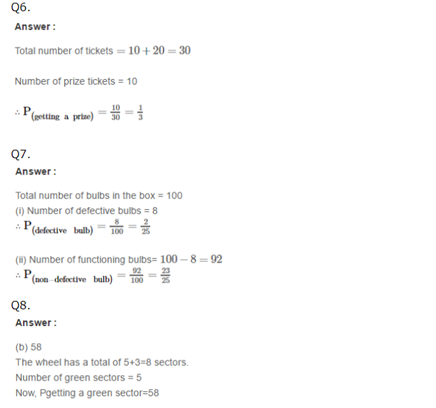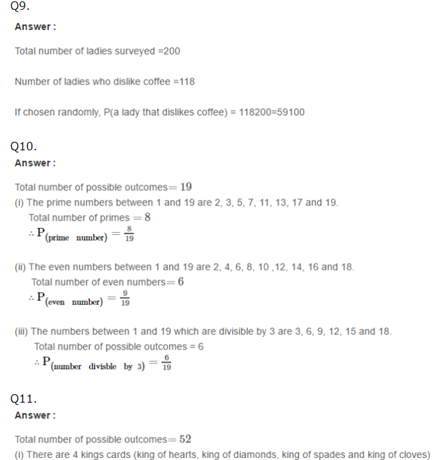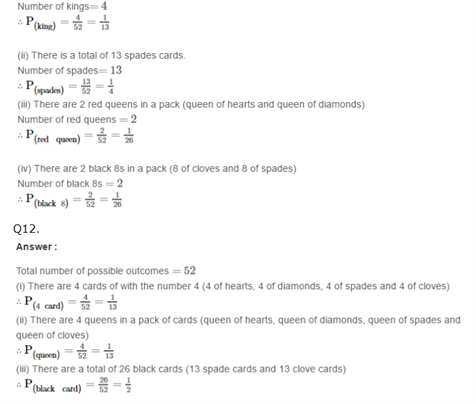Practise This Question

Which of the following statements are correct regarding probiotics?

i. Probiotics restore bacteria present in the intestine.

ii. Probiotics cause different diseases.

iii. Probiotics improve immune health.

iv. Probiotics can be taken in food.